# Civil Engineering - Theory of Structures

Exercise : Theory of Structures - Section 1
6.
A material which obeys Hook's law, is subjected to direct stress σ0. At its elastic limit, the following statement is true,
Strain is equal to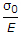Maximum shear stress =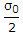Strain energy =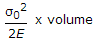Shear strain energy =All the above.
Explanation:
No answer description is available. Let's discuss.

7.
In the truss shown in the given figure, the force in member BC is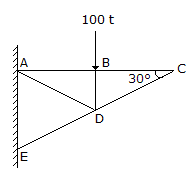100 t compressive
100 t tensile
zero
indeterminate
Explanation:
No answer description is available. Let's discuss.

8.
A concentrated load P is supported by the free end of a quadrantal ring AB whose end B is fixed. The ratio of the vertical to horizontal deflections of the end A, is
π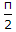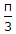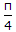Explanation:
No answer description is available. Let's discuss.

9.
Stress may be defined as
force per unit length
force per unit volume
force per unit area
none of these.